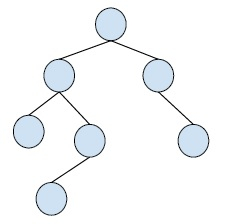# Binary Tree with Array implementation in C++

A binary tree is a special type of tree in which each node of the tree can have at most two child nodes. These child nodes are known as right child and left child.

A simple binary tree is −For representing trees, there are two ways,

• dynamic node representation which uses linked list
• Sequential representation which uses array.

Here, we will discuss about array representation of binary tree. For this we need to number the nodes of the BT. This numbering can start from 0 to (n-1) or from 1 to n.

Lets derive the positions of nodes and their parent and child nodes in the array.

When we use 0 index based sequencing,

Suppose parent node is an index p.

Then, the left_child node is at index (2*p)+ 1.

The right_child node is at index (2*p) + 2.

Root node is at index 0.

left_child is at index 1.

Right_child is at index 2.

When we use 1 index based sequencing,

Suppose parent node is at index p,

Right_node is at index (2*p).

Left_node is at index (2*p)+1.

Root node is at index 1.

left_child is at index 2.

Right_child is at index 3.

## Example

Live Demo

#include<bits/stdc++.h>
using namespace std;
char tree;
int rootnode(char key){
if(tree != '\0')
else
tree = key;
return 0;
}
int leftchild(char key, int parent){
if(tree[parent] == '\0')
cout <<"\nCan't set child at"<<(parent * 2) + 1<<" , no parent found";
else
tree[(parent * 2) + 1] = key;
return 0;
}
int rightchild(char key, int parent){
if(tree[parent] == '\0')
cout<<"\nCan't set child at"<<(parent * 2) + 2<<" , no parent found";
else
tree[(parent * 2) + 2] = key;
return 0;
}
int traversetree(){
cout << "\n";
for(int i = 0; i < 10; i++){
if(tree[i] != '\0')
cout<<tree[i];
else
cout<<"-";
}
return 0;
}
int main(){
rootnode('A');
rightchild('C', 2);
leftchild('D', 0);
rightchild('E', 1);
rightchild('F', 2);
traversetree();
return 0;
}

## Output

Can't set child at6 , no parent found
Can't set child at6 , no parent found
AD--E-----